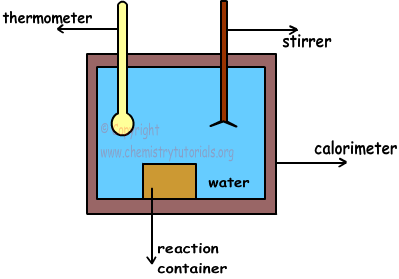# Measuring Enthalpy and Calorimeter

Measuring Enthalpy and Calorimeter

Most of enthalpy change can be measured experimentally. This process is called “measuring heat transfer” calorimetry. Calorimeters are devices used in measuring heat flow. In calorimeters;

Heat Absorbed = Heat Released

A simple calorimeter is shown in picture below;Calorimeter is a container filled with water and insulated.Since it is isolated, there is no heat lost or transfer with surrounding. Matters are placed into the reaction container. With the help of stirrer, we make temperature of water same at everywhere. Moreover, thermometer is used to measure temperature of water before and after reaction completed.

Ti= initial temperature (Before reaction)

Tf=final temperature (After reaction)

After measurements if;

• Ti < Tf, reaction gives heat to water and make increase its temperature. Thus, it is exothermic reaction.
• Ti > Tf, reaction absorbs heat from water and make decrease its temperature. Thus, it is endothermic reaction.

Heat flow in calorimeter is calculated with following formula;

Q=mcal.ccal.∆T + mwater. cwater.∆T

Where;

mcal= mass of calorimeter, in g.

ccal=specific heat capacity of calorimeter

mwater= mass of water in g.

cwater= specific heat capacity of water

∆T= difference between initial and final temperature

Since ∆T is same for water and calorimeter, formula becomes;

Q=(mcal .ccal + mwater + cwater). ∆T

If we write;

Ccal=mcal.ccal

Cwater=mwater.cwater

Q=(Ccal + Cwater). ∆T=Csystem. ∆T

We know number of mols of reactants. According to coefficient of matters in reaction, ∆H value is calculated. If temperature of system increases then, reaction is endothermic, we take ∆H positive. If temperature of system increases then reaction is exothermic and ∆H becomes negative.

Example: Which ones of the following applications are exothermic?

I. X(g) + Y(g) → Z(g) + T(g)

Sum of products’ bond energies is larger than sum of bond energies of reactants.

II. When compound A dissolves in water, temperature of water decreases.

III. 2B(g) + C(g) → 2D(g)

Reaction given above takes place in insulated container and pressure in the container increases.

Solution:

I. If sum of bond energies of products is larger than bond energies of reactants, energy released from forming new bond in products is larger than the energy used to break bonds of reactants. In other words, reaction is exothermic.

II. If temperature of water decrease, then reaction absorbs heat from water. Thus, reaction is endothermic.

III. In this reaction moles of gases decreases, on the contrary pressure increases. To increase pressure, temperature of system also increase. So, reaction must be exothermic.

Example: Combustion enthalpy of coal is -5500 kcal/g. To increase 5 kg water in calorimeter from 20 0C to 42 0C, how many kg of coal must be burned? (cwater=1cal/g 0c)

Solution:

Heat required to increase temperature of 5 kg =5000 g water from 20 0C to 42 0C is calculated with following formula;

Q= m.c.∆T

Q=5000.1.(42-20)

Q=110000 cal

If 1 g coal is burned   5500 cal heat is released

If X g coal is burned  110000 cal heat is released

¯¯¯¯¯¯¯¯¯¯¯¯¯¯¯¯¯¯¯¯¯¯¯¯ ¯¯¯¯¯¯ ¯¯¯

X=20 g coal

Enthalpy of the reactions depends on;

• Quantity of matter
• Physical state of matter
• Pressure
• Temperature
• Types of matter

Thermochemistry Exams and Problem Solutions